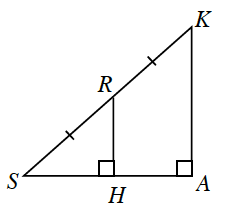### Home > INT2 > Chapter 7 > Lesson 7.1.4 > Problem7-51

7-51.Examine the diagram at right.

1. Are the triangles in this diagram similar? Justify your answer using similarity transformations.

Draw $ΔSHR$ and $ΔSAK$ separately. Which angles are congruent?

2. What is the relationship between the lengths $HR$ and $AK$? Between the lengths $SH$ and $SA$? Between the lengths $SH$ and $HA$?

Compare $SR$ with $SK$. How are these lengths related? If the triangles are similar,
then this relationship will be true for the other pairs of corresponding sides.

$S K = 20 \text { units and } R H = 8 \text { units, what is } H A ?$

3. If $SK = 20$ units and $RH = 8$ units, what is $HA$?

Add the lengths to your diagram from part (a). If $SK = 20$, how long is $SR$?
Use the Pythagorean Theorem to calculate the unknown side length.